# Free associative algebra

(diff) ← Older revision | Latest revision (diff) | Newer revision → (diff)

The algebra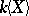of polynomials over a fieldin non-commuting variables in. The following universal property determines the algebrauniquely up to an isomorphism: There is a mapping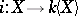such that any mapping frominto an associative algebrawith a unit overcan be factored throughin a unique way. The basic properties ofare:

1)can be imbedded in a skew-field (the Mal'tsev–Neumann theorem);

2)has a weak division algorithm, that is, the relation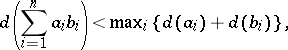where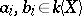, all the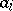are non-zero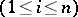,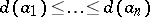, always implies that there are an integer,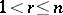, and elements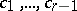such thatand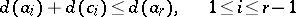(here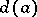is the usual degree of a polynomial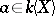,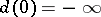);

3)is a left (respectively, right) free ideal ring (that is, any left (respectively, right) ideal ofis a free module of uniquely determined rank);

4) the centralizer of any non-scalar element of(that is, the set of elements that commute with a given element) is isomorphic to the algebra of polynomials overin a single variable (Bergman's theorem).

How to Cite This Entry:
Free associative algebra. Encyclopedia of Mathematics. URL: http://encyclopediaofmath.org/index.php?title=Free_associative_algebra&oldid=16680
This article was adapted from an original article by L.A. Bokut (originator), which appeared in Encyclopedia of Mathematics - ISBN 1402006098. See original article